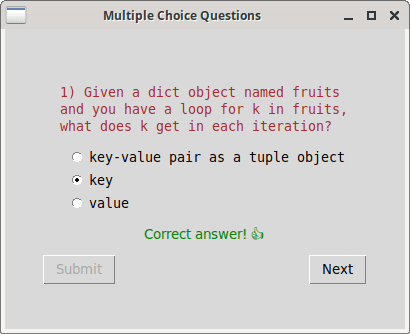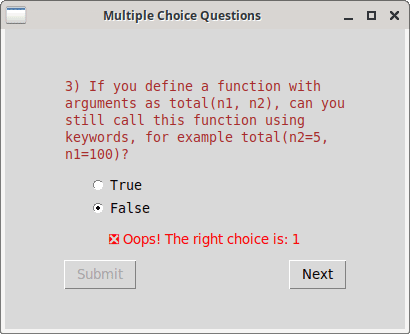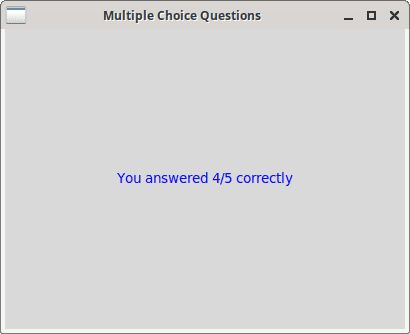# MCQ GUI

In this section, you'll implement a GUI for evaluating multiple choice questions. This will reuse some of the code already presented in earlier sections. The main change from `input()` function implementation is that the user can select and change their choice as many times as they want. The answer would be recorded only when a button is clicked. Another difference is that the questions are asked one at a time, easier to implement here since you have total control over the display screen.

## Code and explanations

Here's one possible implementation:

``````# mcq_gui.py
import tkinter as tk
import random

class Root(tk.Tk):
def __init__(self, question_blocks):
super().__init__()

self.question_blocks = question_blocks
self.q_total = len(self.question_blocks)
self.q_count = 1
self.a_count = 0
self.title('Multiple Choice Questions')
self.geometry('400x300')
self.create_frame()

def create_frame(self):
self.frame = tk.Frame()
self.frame.pack(expand=True)

self.l_info.pack()

self.b_submit = tk.Button(self.frame, text='Submit',
state='disabled', command=self.submit)
self.b_submit.pack(side=tk.LEFT)
self.submit_clicked = False

self.b_next = tk.Button(self.frame, text='Next',
state='disabled', command=self.next)
self.b_next.pack(side=tk.RIGHT)

question, *choices = self.question_blocks[self.q_count-1].split('\n')
random.shuffle(choices)
for idx, self.choice in enumerate(choices, 1):
if self.choice.startswith('--> '):
self.choice = self.choice[4:]
self.choice = self.choice[self.choice.find(" ")+1:]

if not self.submit_clicked:
self.b_submit['state'] = 'normal'

def submit(self):
self.submit_clicked = True
self.a_count += 1
self.l_info['fg'] = 'green'
else:
self.l_info['fg'] = 'red'
self.l_info['text'] = ('\u274E Oops! '
self.b_submit['state'] = 'disabled'
self.b_next['state'] = 'normal'

def next(self):
self.frame.destroy()
self.q_count += 1
if self.q_count <= self.q_total:
self.create_frame()
else:
self.frame = tk.Frame()
self.frame.pack(expand=True)
report = f'You answered {self.a_count}/{self.q_total} correctly'
self.l_report = tk.Label(self.frame, fg='blue', text=report)
self.l_report.pack()

if __name__ == '__main__':
random.shuffle(question_blocks)

root = Root(question_blocks)
root.mainloop()
``````

Most of the widget creation and code logic should be familiar to you from the previous sections. Here's some details specific to this program:

• `wraplength` is used to force a long question into multiple lines.
• `TkFixedFont` (monospace) is used because there are code snippets in some of the questions and answers.
• The `Submit` and `Next` buttons are initially in the `state='disabled'` option. After the user selects one of the choices, the state is changed to `normal` for the `Submit` button.
• To prevent the user from submitting an answer multiple times, a flag is used.
• Once an answer is submitted, the state is changed to `normal` for the `Next` button.
• When the `Next` button is clicked, the current frame is destroyed. If there are pending questions, they are displayed and the process repeats. Else, a final frame shows the report card.

## Screenshots

Here's some screenshots: